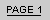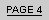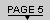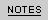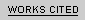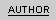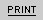I N   S E A R C H   O F   M A S C U L I N I T YP a g e   F i v e :   J o e   B u c k   a n d   R a t s o   R i z z o "I   d o n ' t   t h i n k   I   c a n  w a l k   a n y m o r e , "   s a y s   R a t s o   t o   J o e .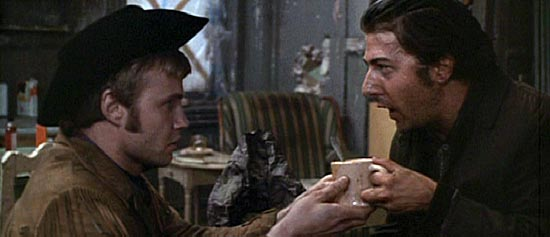A R T I C L E   B Y   A N N   B A R R O W